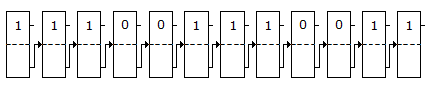# Electronics and Communication Engineering - Digital Electronics

41.

Karnaugh map is used to

 A. minimise the number of flip flops in a digital circuit B. minimise the number of gates only in a digital circuit C. minimise the number of gates and fan in a digital circuit D. design gates

Answer: Option B

Explanation:

No answer description available for this question. Let us discuss.

42.

The main advantage of CMOS circuit is

 A. high gain B. high output impedance C. low power consumption D. high gain and high output impedance

Answer: Option C

Explanation:

No answer description available for this question. Let us discuss.

43.

Floating gate is fabricated for making MOS

 A. ROM B. PROM C. EPROM D. EAROM

Answer: Option C

Explanation:

No answer description available for this question. Let us discuss.

44.

A 12-bit binary counter has the following state. The octal number represented isA. 677 B. 7163 C. 7128 D. 7183

Answer: Option B

Explanation:

No answer description available for this question. Let us discuss.

45.

(1111.11)2 is

 A. (1.01)10 B. (0.75)10 C. (15.3)10 D. (15.75)10

Answer: Option D

Explanation:

No answer description available for this question. Let us discuss.

#### Current Affairs 2021

Interview Questions and Answers